## Introduction

The spcosa-package implements algorithms for spatial coverage sampling and for random sampling from compact geographical strata based on the k-means algorithm (de Gruijter et al., 2006, Walvoort et al., 2010). Spatial coverage sampling is known to be an efficient sampling method for model-based mapping (kriging). Random sampling from compact geographical strata is recommended for design-based estimation of spatial means, proportions, etc.. In this vignette, the usage of the package will be demonstrated by means of several examples. In addition to spatial coverage sampling also stratified simple random sampling of compact strata will be treated in this vignette.

The spocsa-package can be attached by means of

library(spcosa)

Loading required package: rJava


In addition, we will also attach the ggplot2 package for modifying plots:

library(ggplot2)


Although the implemented optimisation algorithms are deterministic in nature, they use a user-specified number (nTry, see examples below) of random initial configurations to reduce the risk of ending up in an unfavourable local optimum. In order to be able to reproduce the sampling patterns in a later stage, the pseudo random number generator of R has to be initialised first:

set.seed(314)


The spcosa-package depends on the sp-package (Pebesma & Bivand, 2005) for storing spatial information, and the ggplot2-package (Wickham, 2009) for visualisation. A basic knowledge of the sp-package is highly recommended. Knowledge of the ggplot2-package is only needed for fine-tuning spcosa graphics. Consult the superb ggplot2-website for details and illustrative examples.

## Algorithms

The basic idea is to distribute sampling points evenly over the study area by selecting these points in compact spatial strata. Compact strata can be obtained by k-means clustering of the cells making up a fine grid representing the study area of interest. Two k-means algorithms have been implemented in the spcosa-package: a transfer algorithm and a swopping algorithm (Walvoort et al, 2010). The transfer algorithm obtains compact clusters (strata) by transferring cells from one cluster to the other, whereas the swopping algorithm achieves this by swopping cells between clusters. The first algorithm results in compact clusters, whereas the second algorithm results in compact clusters of equal size. For reasons of efficiency, both algorithms have been implemented in the Java language and communicate with R (R Core Team, 2015) by means of the rJava-package (Urbanek, 2013).

## Examples

In this section, the spcosa-package will be demonstrated by means of several examples.

### Spatial coverage sampling without prior points

In the first example, spatial coverage sampling will be applied to map clay content in a study area by means of ordinary point kriging.

First, a vector or raster representation of the study area has to be loaded. As an example, the ASCII-grid demoGrid.asc residing in the maps directory of the spcosa-package will be read by means of the readGDAL-function of the rgdal-package (Bivand et al., 2015)

library(rgdal)
filename <- system.file("maps", "demoGrid.asc", package = "spcosa")
ascii_grid <- readGDAL(fname = filename, silent = TRUE)


To obtain a uniform distribution of sampling points over the study area, the sampling points will be selected at the centroids of compact spatial strata. Compact strata can be constructed by invoking the stratify method:

stratification <- stratify(ascii_grid, nStrata = 75, nTry = 10)


In this example, the study area has been partitioned into 75 compact strata. The resulting stratification can be plotted by means of:

plot(stratification)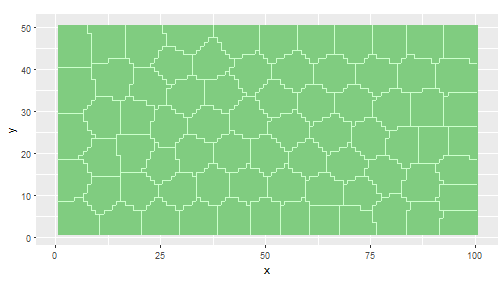Each plot can be modified by adding ggplot2-functions to the plot-method:

plot(stratification) +
scale_x_continuous(name = "Easting (km)") +
scale_y_continuous(name = "Northing (km)")

Scale for 'x' is already present. Adding another scale for 'x', which
will replace the existing scale.

Scale for 'y' is already present. Adding another scale for 'y', which
will replace the existing scale.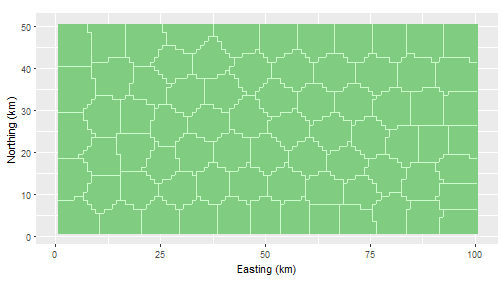The spsample-method, an overloaded method from the sp-package, can be used to select the centroid of each stratum:

sampling_pattern <- spsample(stratification)


The plot-method can be used to visualise the resulting sampling pattern:

plot(sampling_pattern)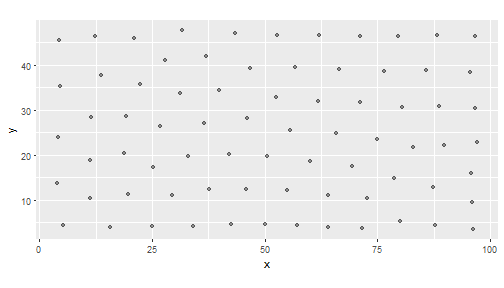The sampling pattern can also be plotted on top of the stratification:

plot(stratification, sampling_pattern)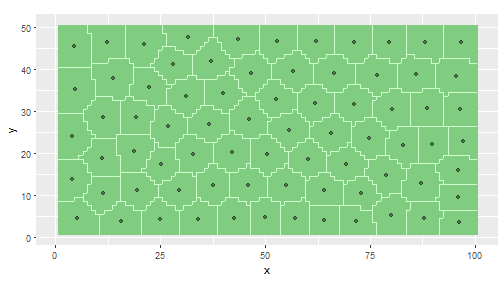Sampling locations can be extracted by means of a simple type cast to class data.frame:

sampling_points <- as(sampling_pattern, "data.frame")

          x         y
1 79.971831  5.253521
2 13.703704 37.802469
3 11.338710 10.435484
4 26.808824 26.323529
5  4.090909 24.038961
6 25.298246 17.298246


Next, field work needs to be done to acquire data at these locations.

Loading required package: gstat


These data will be stored in a data.frame:

head(my_data)

  clay SOM
1  8.0 4.4
2  7.6 4.0
3 13.5 6.9
4 11.7 6.0
5 10.7 5.4
6 11.3 6.1


Model-based inference will be applied to predict clay contents. Model-based inference is not implemented in the spcosa-package, because other add-on packages already provide excellent support for it. See for instance, gstat (Pebesma, 2004), spatial (Venables & Ripley, 2002), and geoR (Diggle & Ribeiro, 2007).

As an example, we will use the gstat package for predicting clay contents by means of ordinary point kriging. First, the observations and sampling pattern will be combined in a single object of class SpatialPointsDataFrame:

#sp_data <- data.frame(sampling_points, my_data)
#coordinates(sp_data) <- ~ x * y
sp_data <- SpatialPointsDataFrame(coords = sampling_points, data = my_data)


Next, the sample variogram for clay has to be estimated, and needs to be fitted by a permissable model:

sample_variogram <- variogram(clay ~ 1, sp_data)
variogram_model <- fit.variogram(sample_variogram,
model = vgm(psill = 1, model = "Exp", range = 5, nugget = 0))
plot(sample_variogram, model = variogram_model)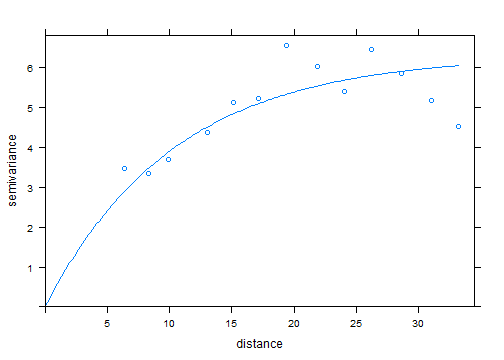Finally, ordinary point kriging predictions can be obtained by means of:

g <- gstat(id = "clay", formula = clay ~ 1, data = sp_data, model = variogram_model)
y_hat <- predict(g, newdata = ascii_grid)

[using ordinary kriging]


The resulting predictions and variances of the prediction error are given in the figures below: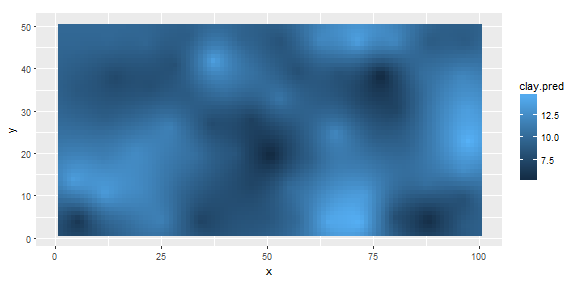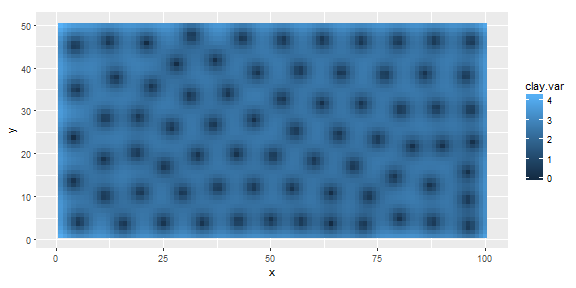### Spatial coverage sampling with prior points

Sometimes, samples from previous sampling campaigns are available. In these situations, spatial infill sampling may be performed. This type of spatial coverage sampling aims to distribute new sampling points evenly over the study area, while taking the locations of existing sampling points into account. Suppose a data.frame is available containing the coordinates of 50 existing sampling points:

head(prior_points)

     x    y
1  9.5 14.6
2 53.1  9.4
3 18.1 38.5
4 56.0 37.6
5 64.6  1.0
6 90.3  1.3


Twenty-five new points can be assigned to sparsely sampled regions by means of:

coordinates(prior_points) <- ~ x * y
stratification <- stratify(ascii_grid, priorPoints = prior_points, nStrata = 75, nTry = 100)
sampling_pattern <- spsample(stratification)


Note that the total number of strata, and therefore the total number of points, equals 50+25=75. In addition, also note that the nTry argument has been set to 100. The algorithm will now use 100 random starting configurations and keeps the best solution to reduce the risk of ending up in an unfavourable local optimum.

plot(stratification, sampling_pattern)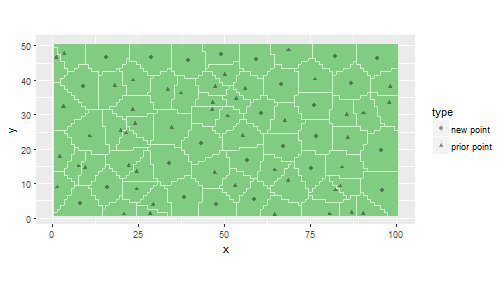Note that prior points and new points are represented by different symbols.

A map of clay contents can be obtained by means of ordinary point kriging. Suppose again, that data have been collected in the field and stored in data.frame sp_data:

Again, we will use gstat for model-based inference:

sample_variogram <- variogram(clay~1, sp_data)
variogram_model <- fit.variogram(sample_variogram,
model = vgm(psill = 1, model = "Exp", range = 1, nugget = 0))
plot(sample_variogram, model = variogram_model)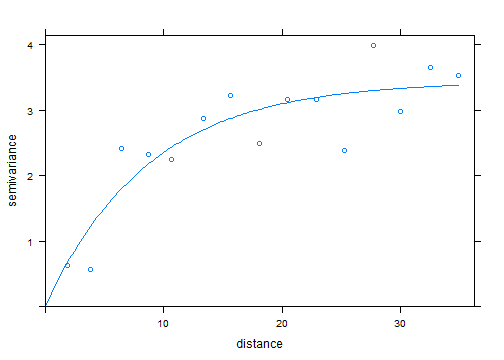g <- gstat(id = "clay", formula = clay ~ 1, data = sp_data, model = variogram_model)
y_hat <- predict(g, newdata = ascii_grid)

[using ordinary kriging]


The resulting predictions and variances of the prediction error are given in the figures below: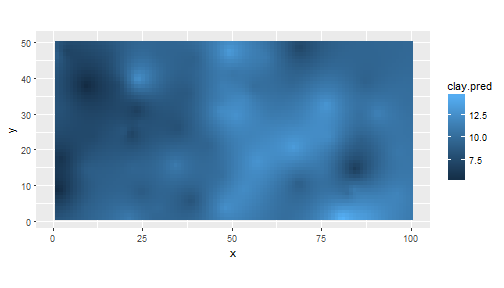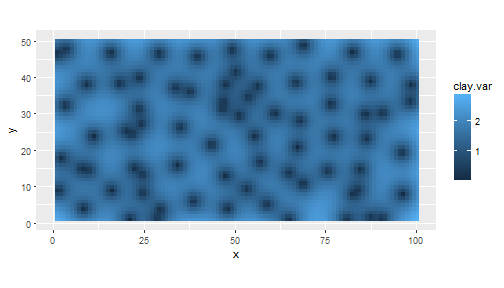### Random sampling from compact geographical strata

In this section, the global mean clay and organic matter contents of the study area will be estimated by means of stratified simple random sampling. Again, we will use ascii_grid as a representation of the study area. The study area will be partitioned into 25 compact strata:

stratification <- stratify(ascii_grid, nStrata = 25, nTry = 10)

plot(stratification)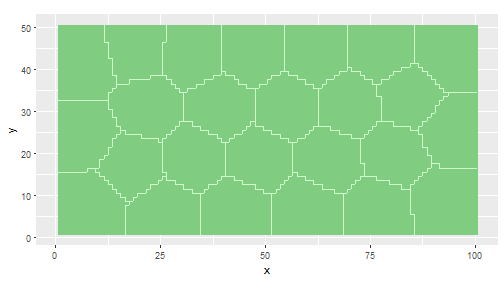The spsample-method can be used to randomly sample two sampling units per stratum:

sampling_pattern <- spsample(stratification, n = 2)

plot(stratification, sampling_pattern)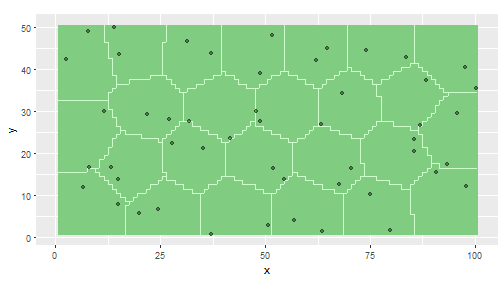Sampling points can be extracted by means of a type cast to class data.frame:

sampling_points <- as(sampling_pattern, "data.frame")

          x         y
1 63.489841  1.449016
2 56.781129  3.942664
3 48.708033 27.649526
4 47.730790 29.879614
5 14.985959  7.704725
6  6.487393 11.906204


Next, some field work has to be done. Again, the results will be stored in data.frame my_data:

The spatial mean clay and soil organic matter contents can be estimated by (de Gruijter et al., 2006):

estimate("spatial mean", stratification, sampling_pattern, my_data)

    clay      SOM
10.20568  5.05320


In estimating the spatial mean, differences in surface area of the strata are taken into account. Note, that the spatial mean is estimated for all columns in my_data. The standard error can be estimated in a similar way:

estimate("standard error", stratification, sampling_pattern, my_data)

     clay       SOM
0.2050475 0.1148747


The spatial cumulative distribution function (SCDF) (see deGruijter et al., 2006) can be estimated by means of

scdf <- estimate("scdf", stratification, sampling_pattern, my_data)


The SCDFs are returned as a list of matrices, i.e. one matrix for each property:

lapply(X = scdf, FUN = head, n = 4)

$clay value cumFreq [1,] 5.0 0.0000 [2,] 6.6 0.0173 [3,] 7.5 0.0385 [4,] 7.8 0.0587$SOM
value cumFreq
[1,]   2.4  0.0000
[2,]   3.0  0.0173
[3,]   3.3  0.0385
[4,]   3.5  0.0587

lapply(X = scdf, FUN = tail, n = 4)

$clay value cumFreq [38,] 13.5 0.9008 [39,] 14.1 0.9412 [40,] 14.7 0.9606 [41,] 15.0 0.9800$SOM
value cumFreq
[27,]   6.5  0.8811
[28,]   6.7  0.9015
[29,]   7.0  0.9406
[30,]   7.1  0.9806


The SCDFs for clay and SOM are visualised below.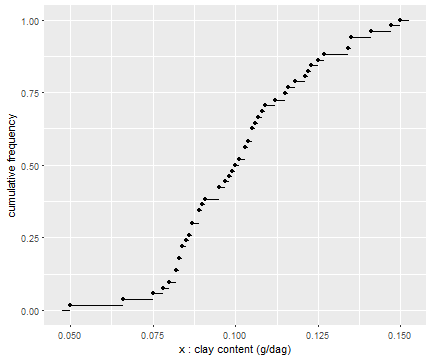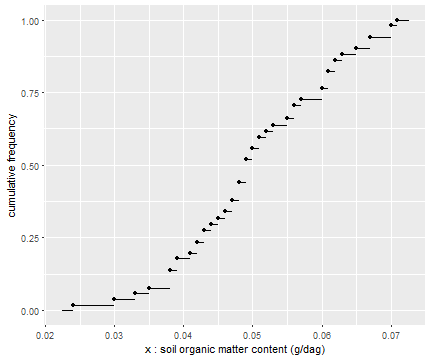### Stratified simple random sampling for composites

In this example, the aim is to estimate the global mean clay and organic matter contents of a field. To reduce laboratory costs, the soil aliquots collected at the sampling locations will be bulked into composite samples. The study area is a field near the village of Farmsum, in the North-East of the Netherlands. An ESRI shape file of this field is available in the maps directory of the spcosa-package. It can be loaded by means of readOGR, a function in the rgdal package (Bivand et al., 2015):

directory <- system.file("maps", package = "spcosa")
shp_farmsum <- readOGR(dsn = directory, layer = "farmsum", verbose = FALSE)


First, the field will be stratified into, say, 20 compact strata of equal size. Strata of equal size are desirable to simplify fieldwork, i.e. equal supports of soil can be collected at the sampling locations (Brus et al., 1999, de Gruijter et al., 2006).

stratification <- stratify(shp_farmsum, nStrata = 20, equalArea = TRUE, nTry = 10)
plot(stratification)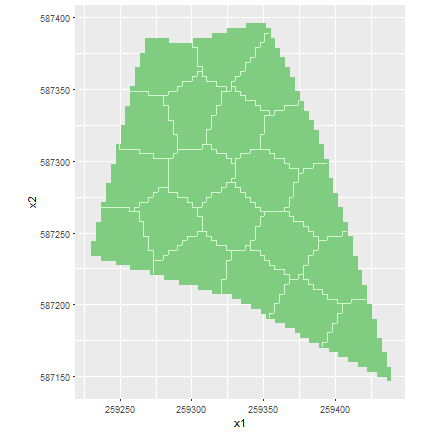Next, two sampling units will be selected at random in each stratum. At least two sampling units per stratum are required to estimate the sampling variance of the estimated mean.

sampling_pattern <- spsample(stratification, n = 2, type = "composite")
plot(stratification, sampling_pattern)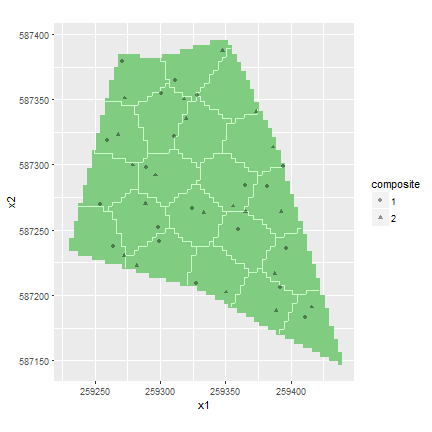Sampling points can be extracted means of:

sampling_points <- as(sampling_pattern, "data.frame")

  composite       x1       x2
1         1 259365.0 587284.3
2         2 259355.6 587268.2
3         1 259324.5 587266.6
4         2 259333.1 587263.4
5         1 259289.5 587298.4
6         2 259296.2 587292.2


Note that an extra column has been added specifying the sampling units to be bulked into each composite. A composite sample is formed by bulking one aliquot (sampling unit) per stratum. Field work now results in a composite sample of size two. These data will be stored in a data.frame called my_data:

The spatial mean and its standard error can be estimated by means of:

estimate("spatial mean", stratification, sampling_pattern, my_data)

clay  SOM
9.05 4.60

estimate("standard error", stratification, sampling_pattern, my_data)

  clay    SOM
0.0225 0.0100


If we do not want to bulk soil aliquots, the same stratification can be used to select a sample of 20 $$\times$$ 2 sampling locations:

sampling_pattern <- spsample(stratification, n = 2)
sampling_points <- as(sampling_pattern, "data.frame")

        x1       x2
1 259354.3 587272.1
2 259355.3 587299.6
3 259325.0 587239.1
4 259312.0 587257.9
5 259324.7 587290.3
6 259309.9 587275.5

plot(stratification, sampling_pattern)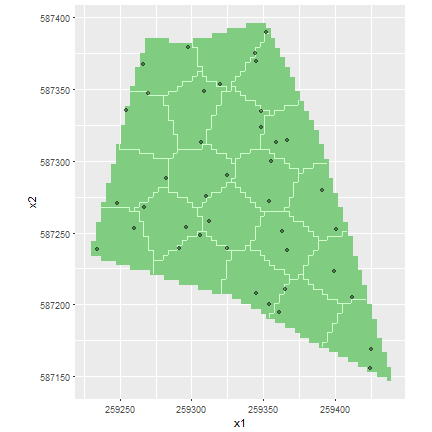## Special cases

### Map projections

In the examples above, the maps didn't have projection attributes. At field scale, projection attributes are not really necessary. However, at continental and global scales, for example, projection attributes can't be ignored. The spcosa-package is capable of handling projection attributes of class CRS. More information on projections is available at the PROJ.4' project website and in the rgdal-package documentation (Bivand et al., 2015).

To illustrate the effect of stratification on smaller spatial scales, consider two (relatively coarse) grids covering the surface of the earth:

grd <- expand.grid(
longitude = seq(from = -176, to = 180, by = 4),
latitude  = seq(from =  -86, to =  86, by = 4)
)
gridded(grd) <-  ~ longitude * latitude

grd_crs <- grd
proj4string(grd_crs) <- CRS("+proj=longlat +ellps=WGS84")


Note that grd is identical to grd_crs, except that grd_crs has projection attributes. If no projection attributes are available, the algorithms in the spcosa-package use squared Euclidean distances in the k-means algorithms. However, if projection attributes have been set, the coordinates will be transformed to lat/long format (latitude/longitude) and squared great circle distances will be used instead.

Both grids will be partitioned into 50 compact geographical strata:

strata     <- stratify(grd,     nStrata = 50)
strata_crs <- stratify(grd_crs, nStrata = 50)

plot(strata)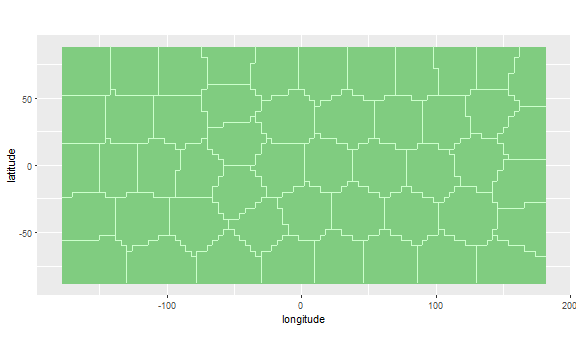plot(strata_crs)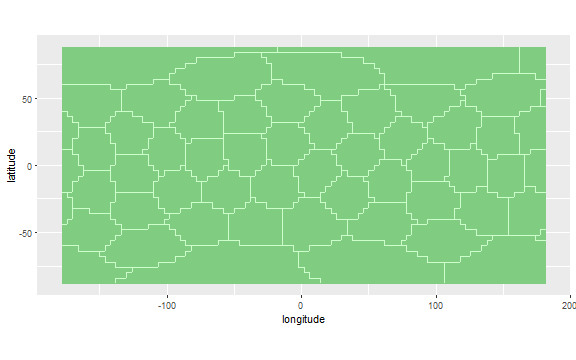Note that grd seems to have more compact strata near the geographic poles than grd_crs. However, the contrary is true. This becomes evident when both stratifications are projected on a sphere (use your mouse to interact with these spheres):